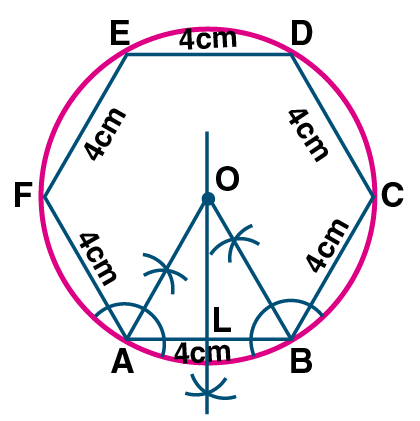# ML Aggarwal Solutions for Class 10 Maths Chapter 16 Constructions

ML Aggarwal Solutions for Class 10 Maths Chapter 16 – Constructions are provided here to help students prepare for their exams and score well. This chapter mainly deals with problems based on constructions. In order to enhance the performance of students in the Class 10 exam, we at BYJU’S have formulated the ML Aggarwal Solutions for Class 10 Maths. Our solution module utilizes various shortcut tips and practical examples to explain all the exercise questions in a simple and easily understandable language and to help students grasp the concepts faster and to make learning fun. These solutions are completely based on the exam-oriented approach to help students score well in their board examination. Practising these questions will ensure that they can easily excel in their final exams. Experts have designed ML Aggarwal Solutions in a very lucid manner that helps students solve problems in the most efficient possible ways. Students can easily download the pdf consisting of this chapter solutions, which are available in the links provided below and can use it for future reference as well.

Chapter 16 – Constructions contains two exercises and the ML Aggarwal Class 10 Solutions present in this page provide solutions to questions related to each exercise present in this chapter.

## ML Aggarwal Solutions for Class 10 Maths Chapter 16 :-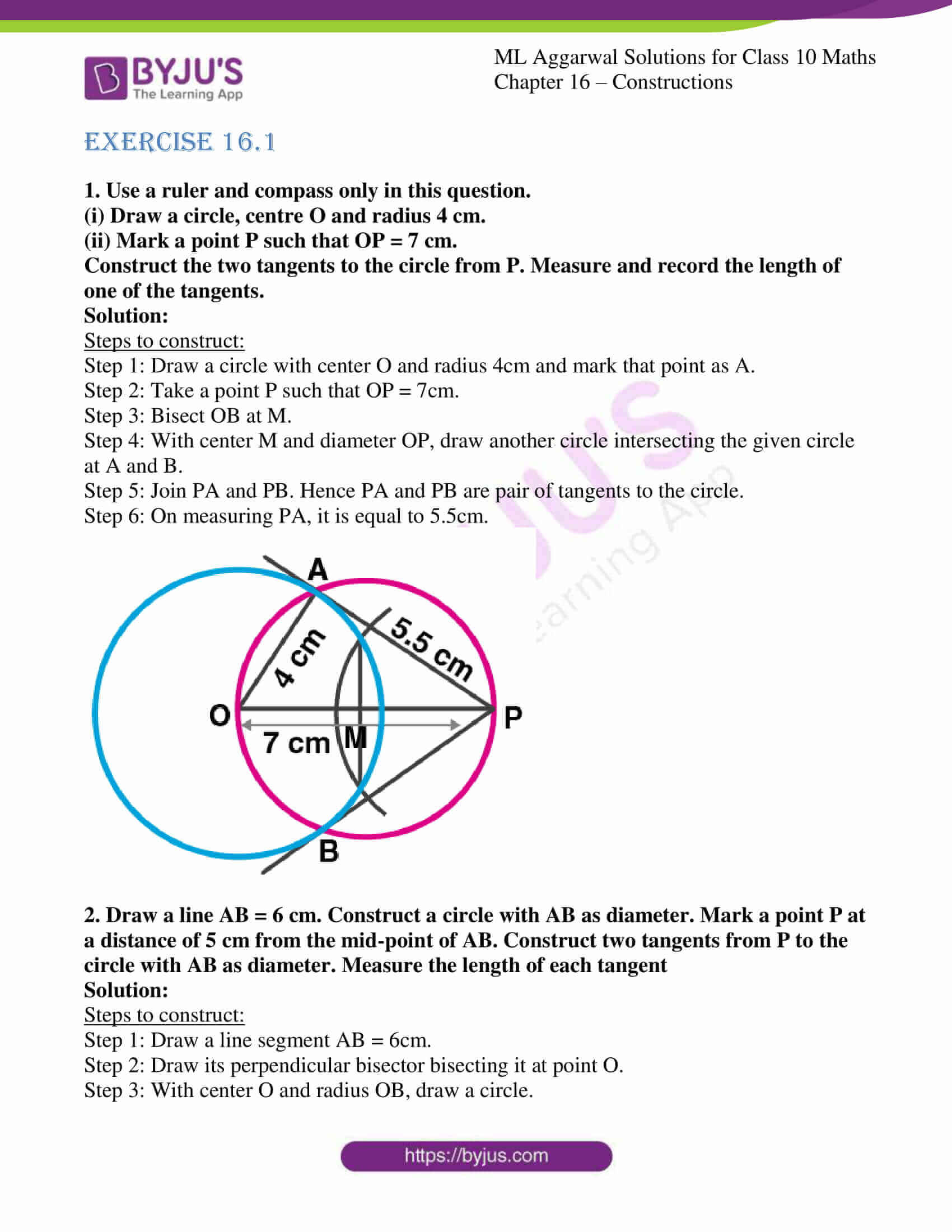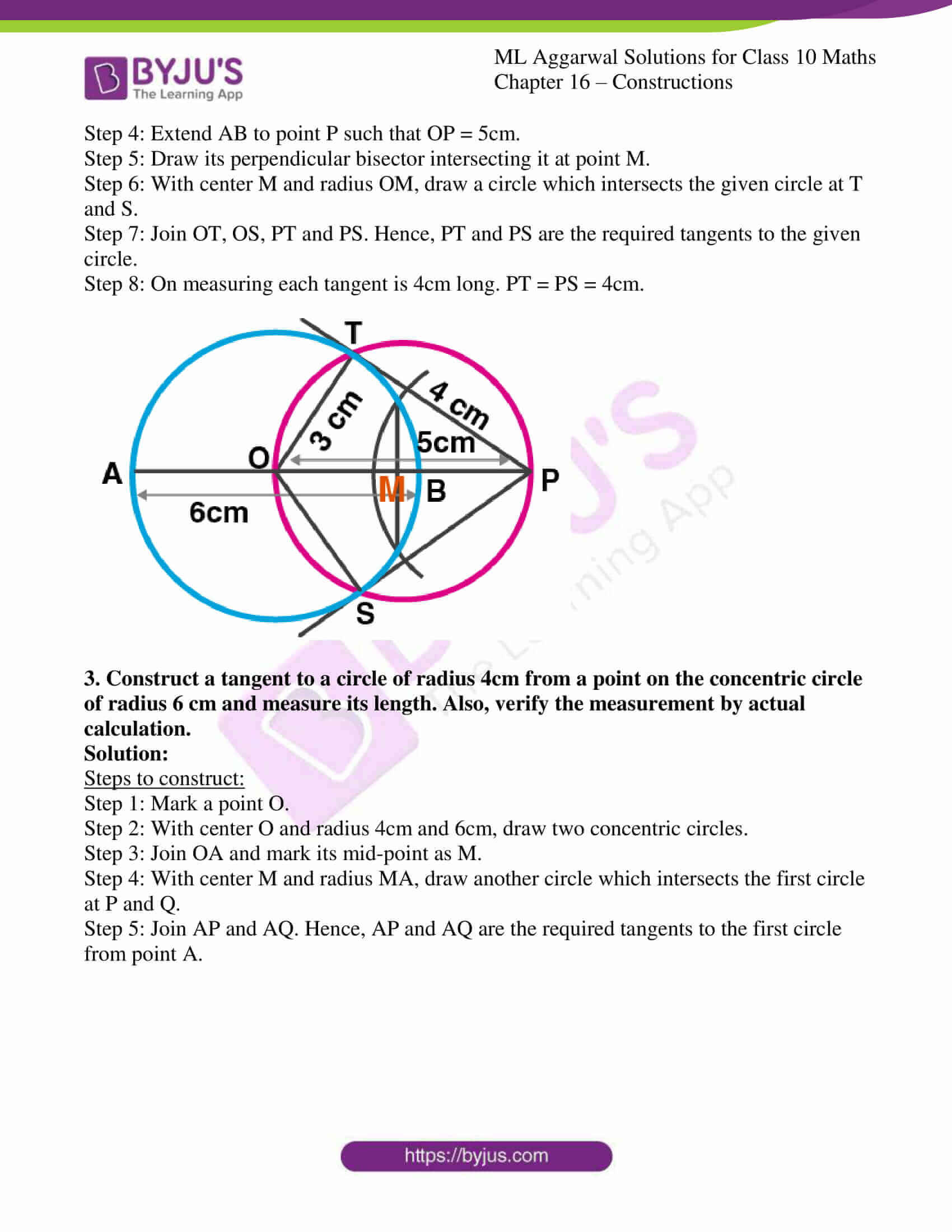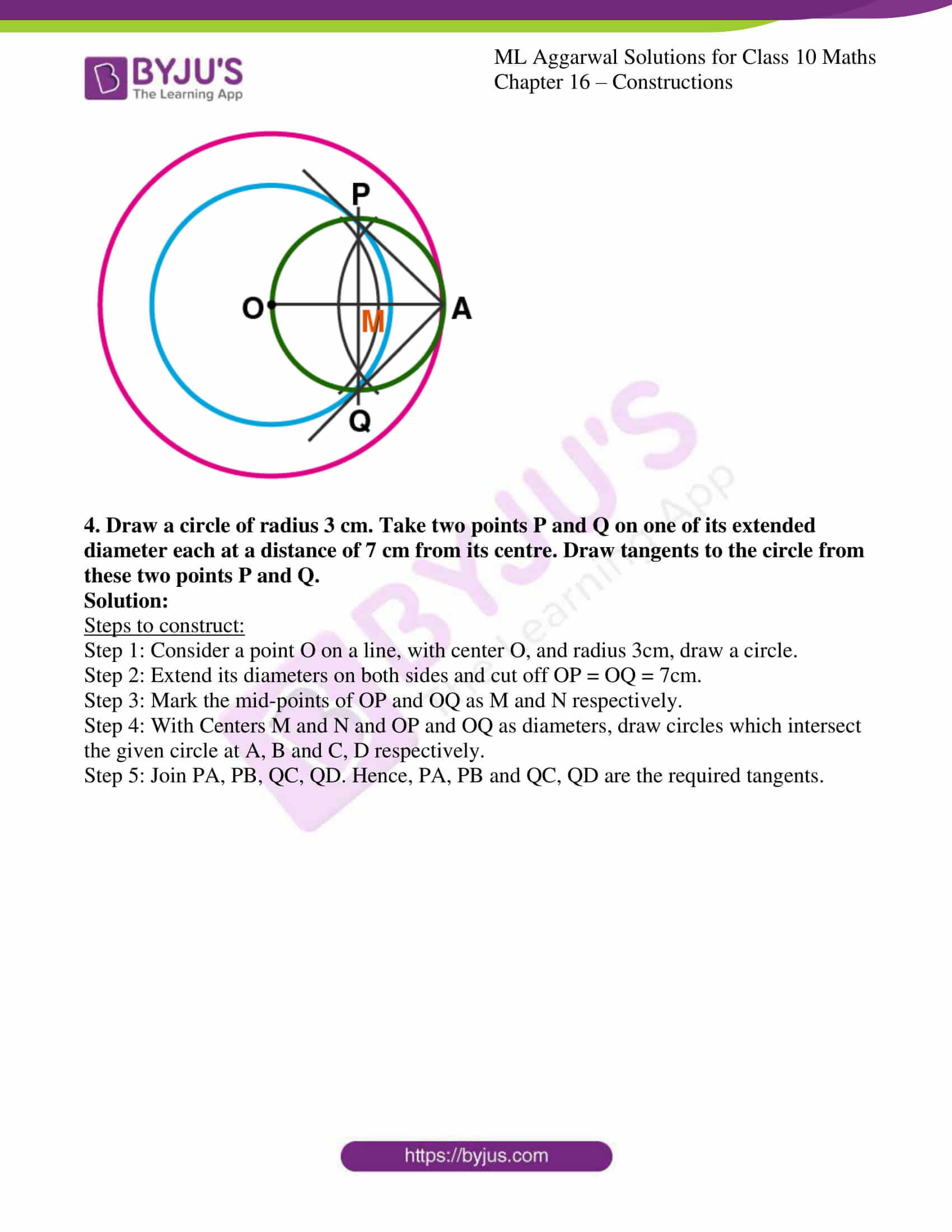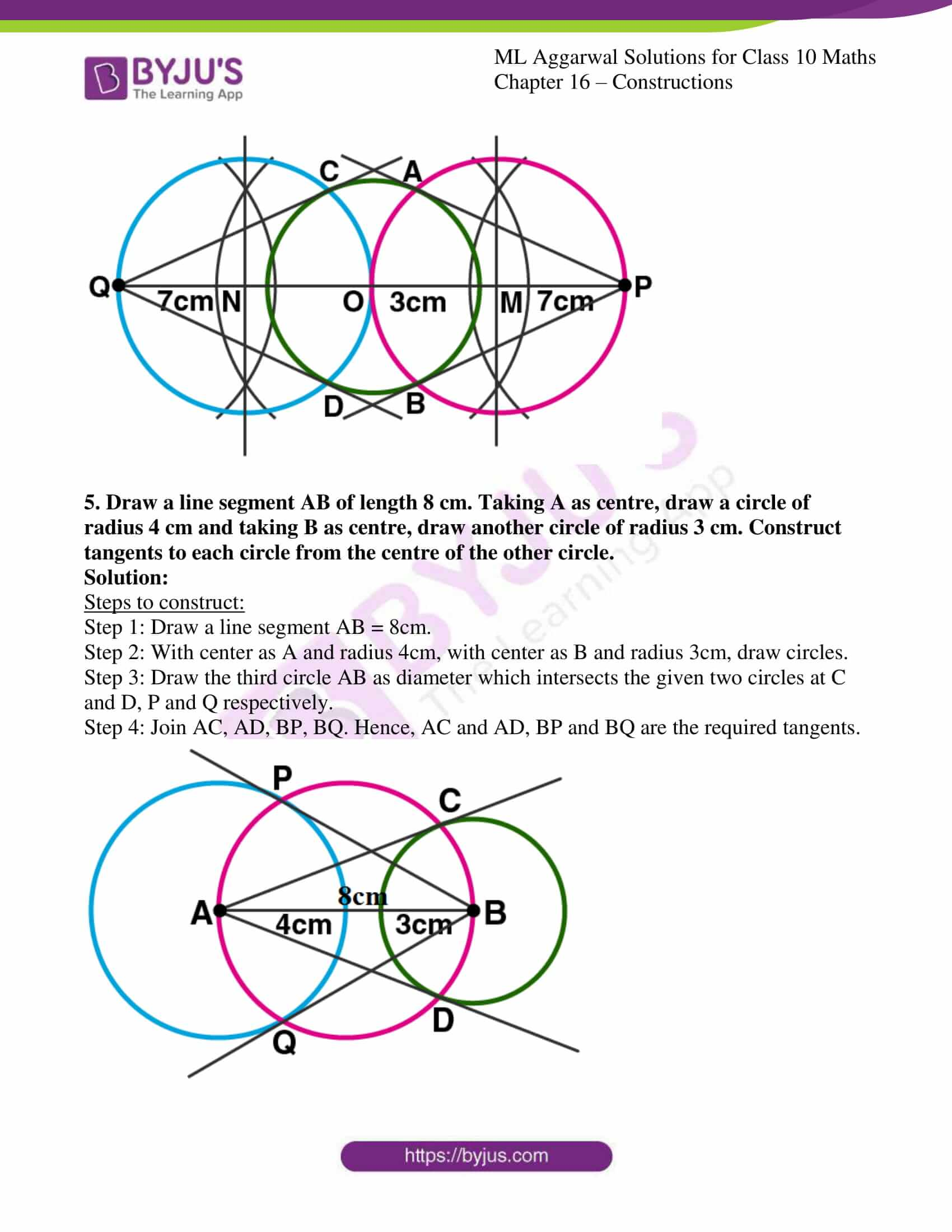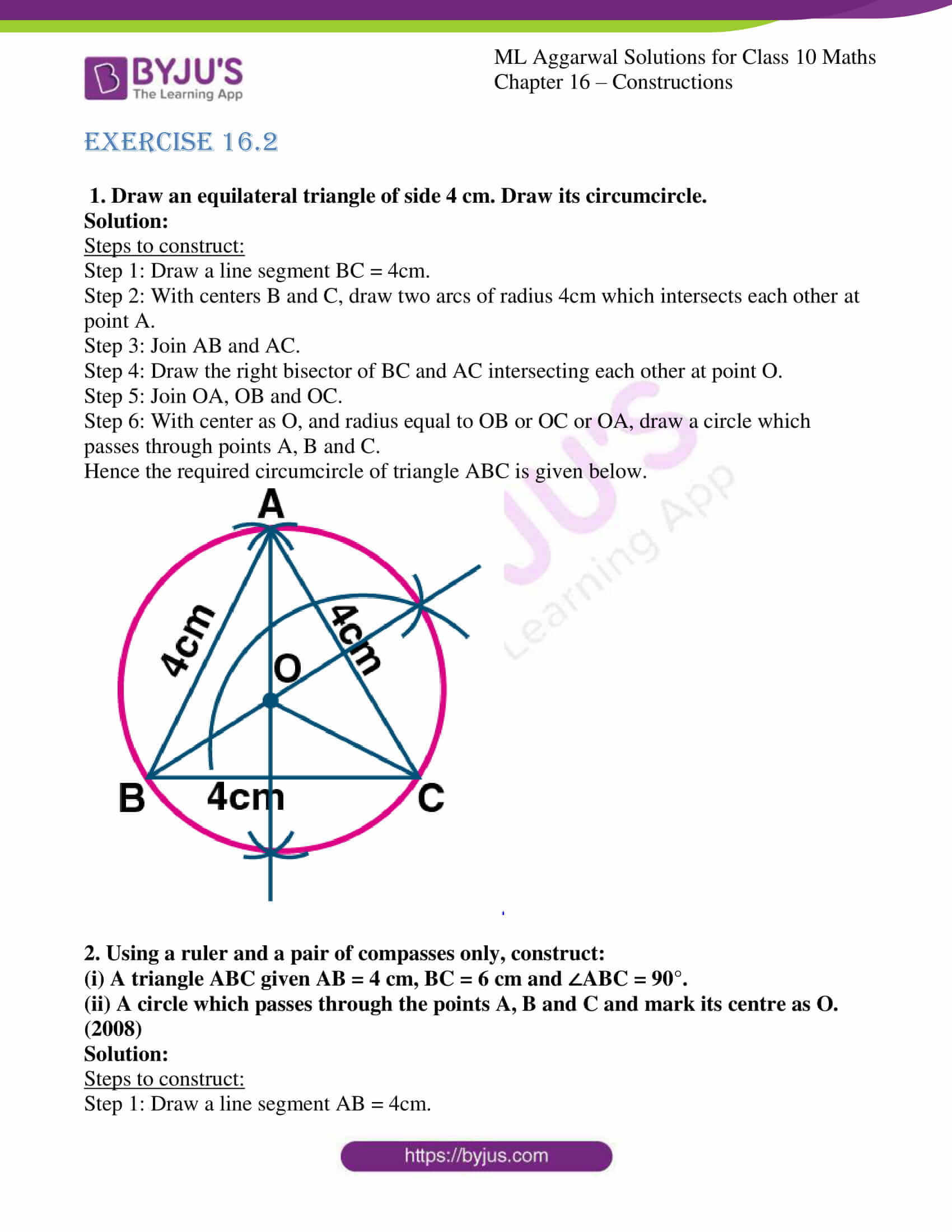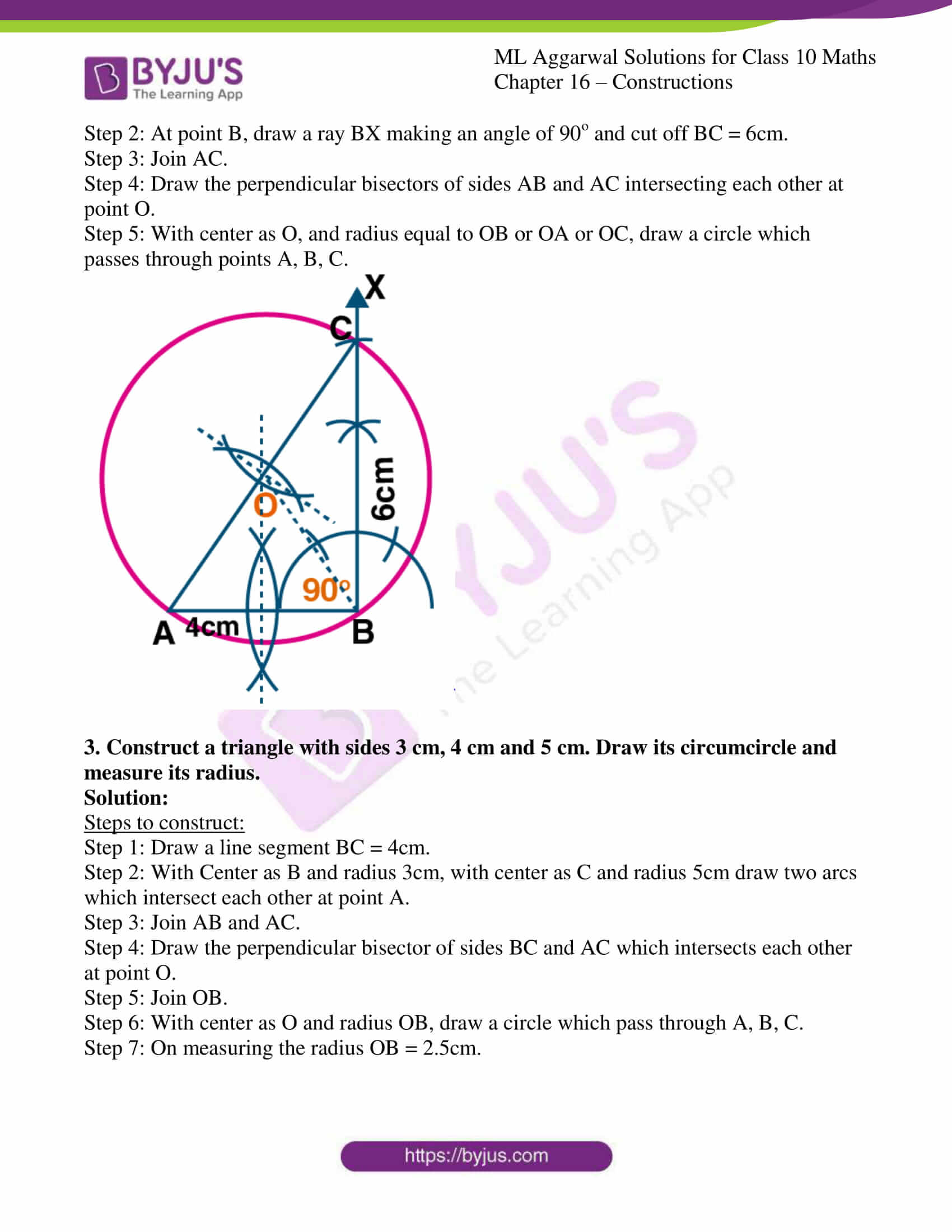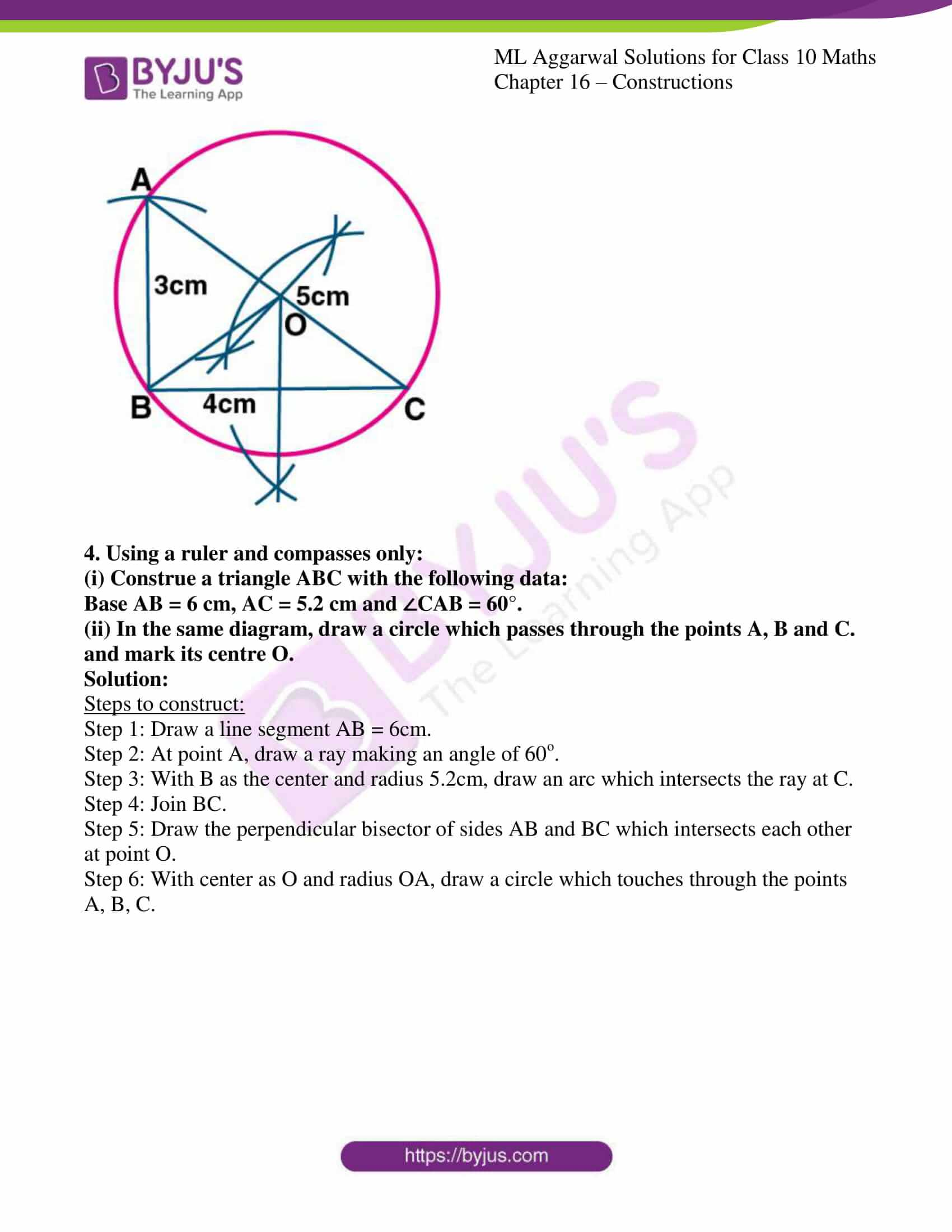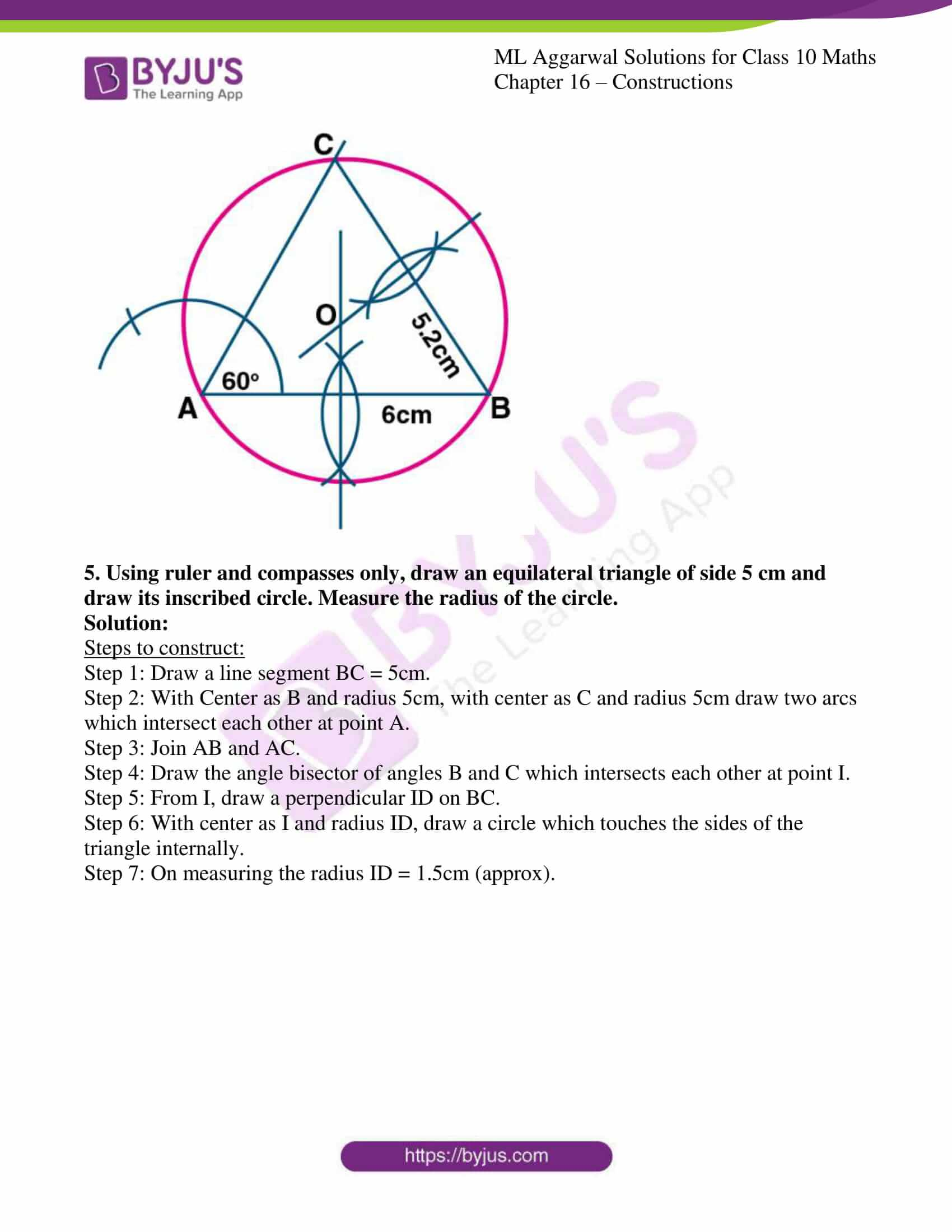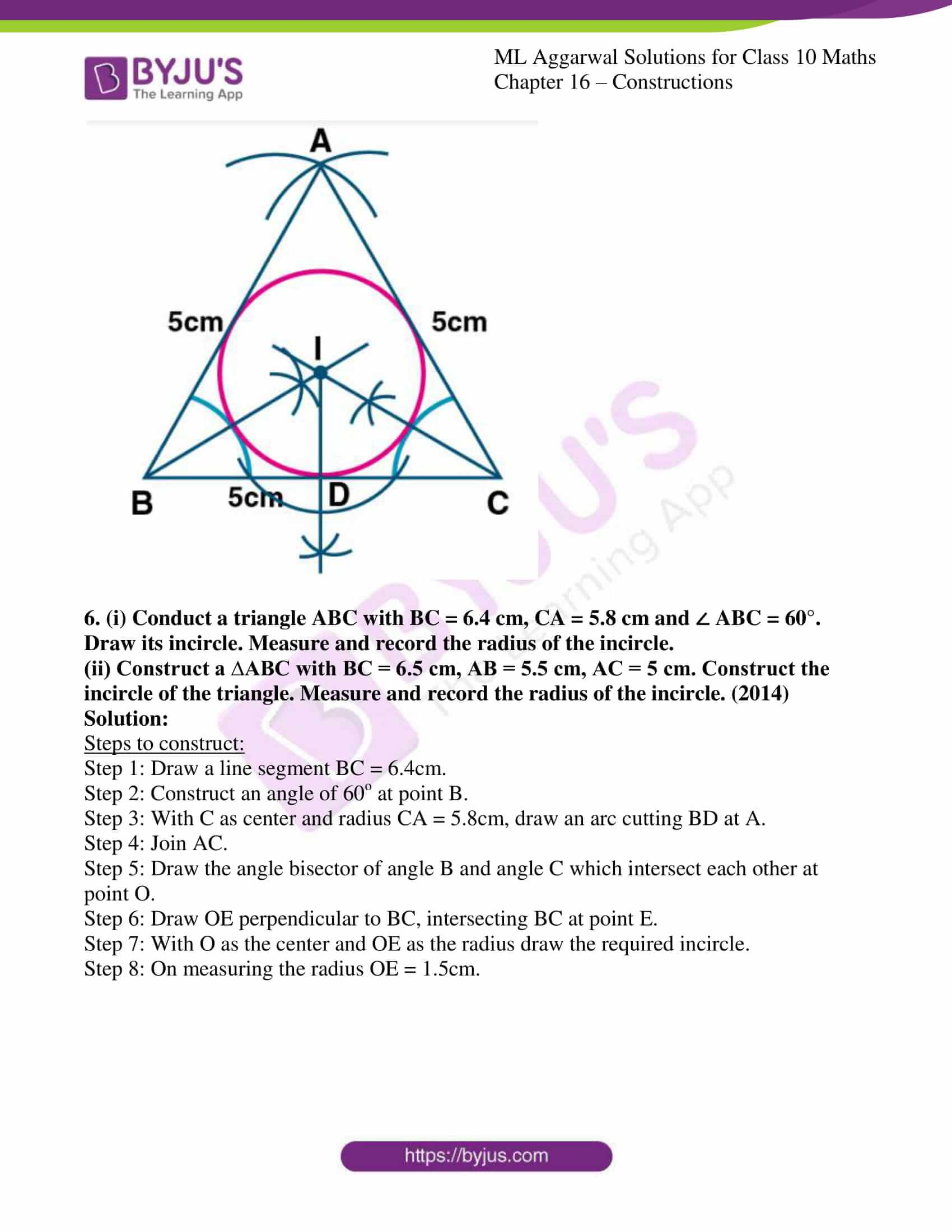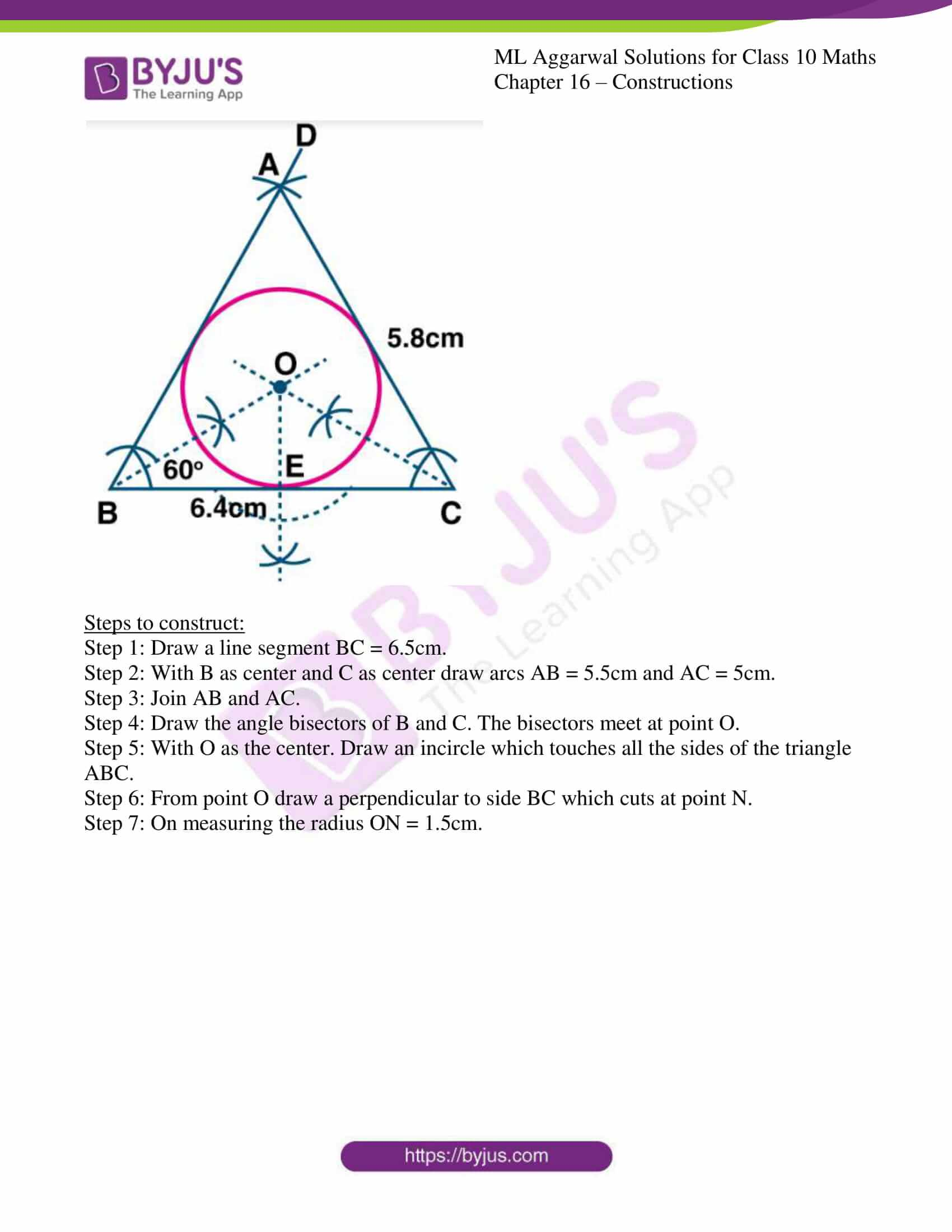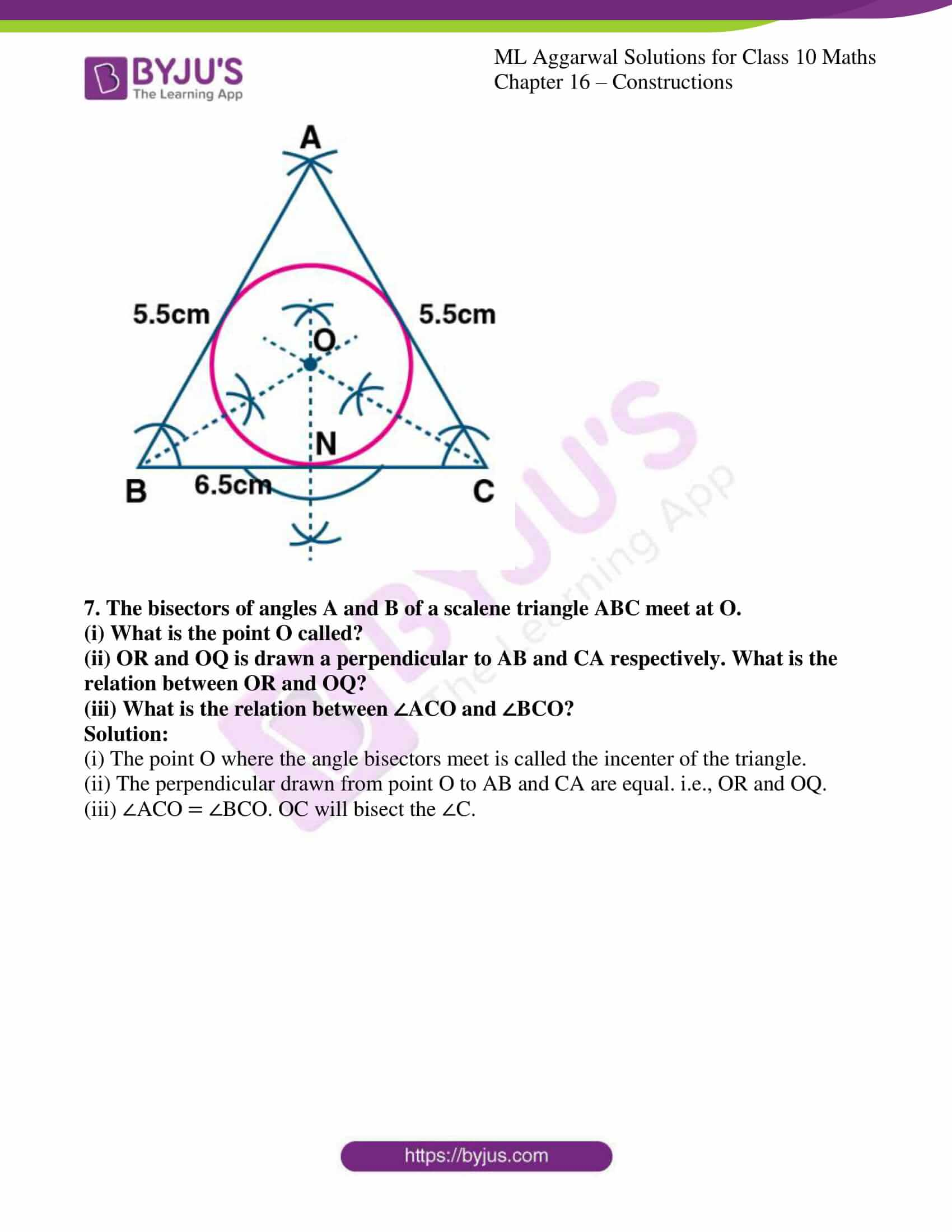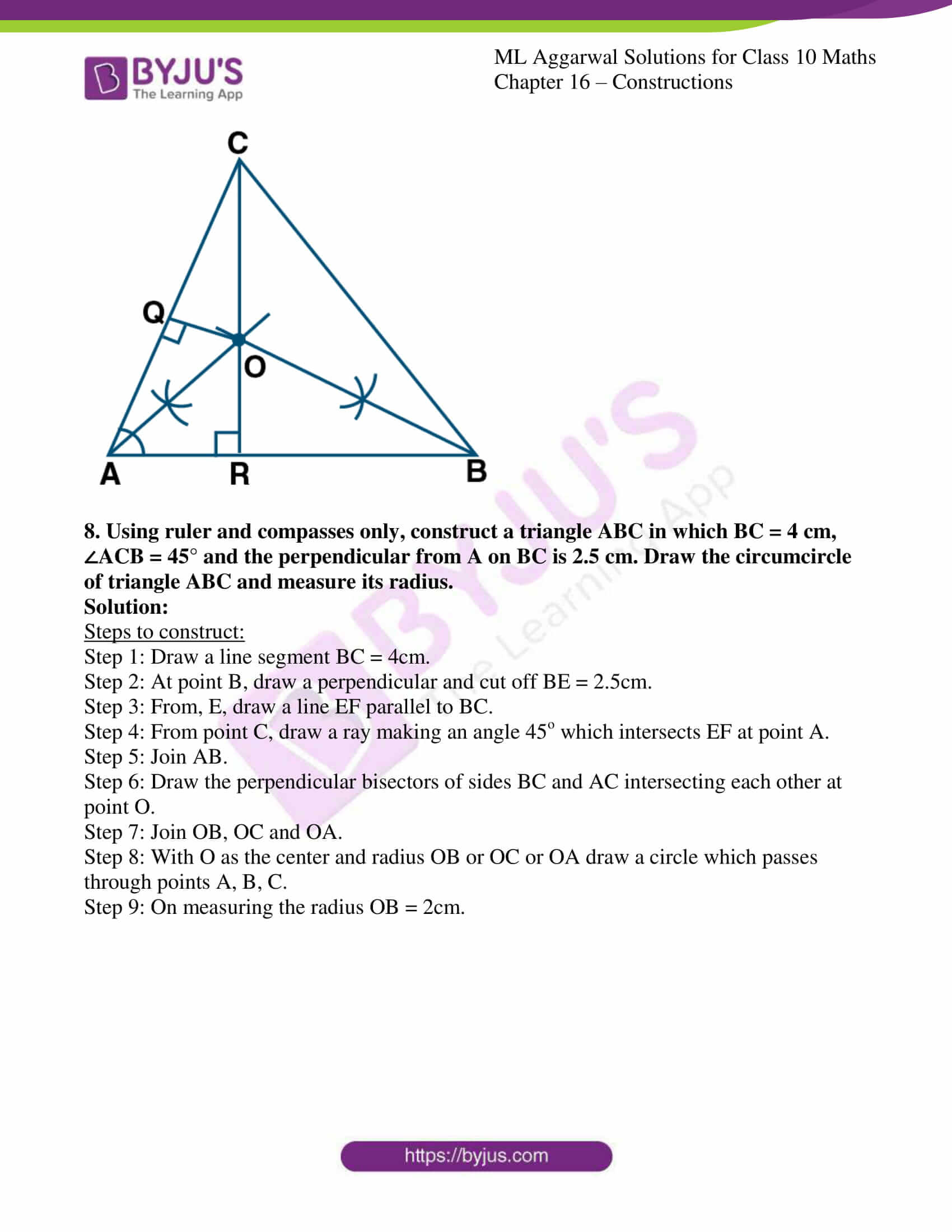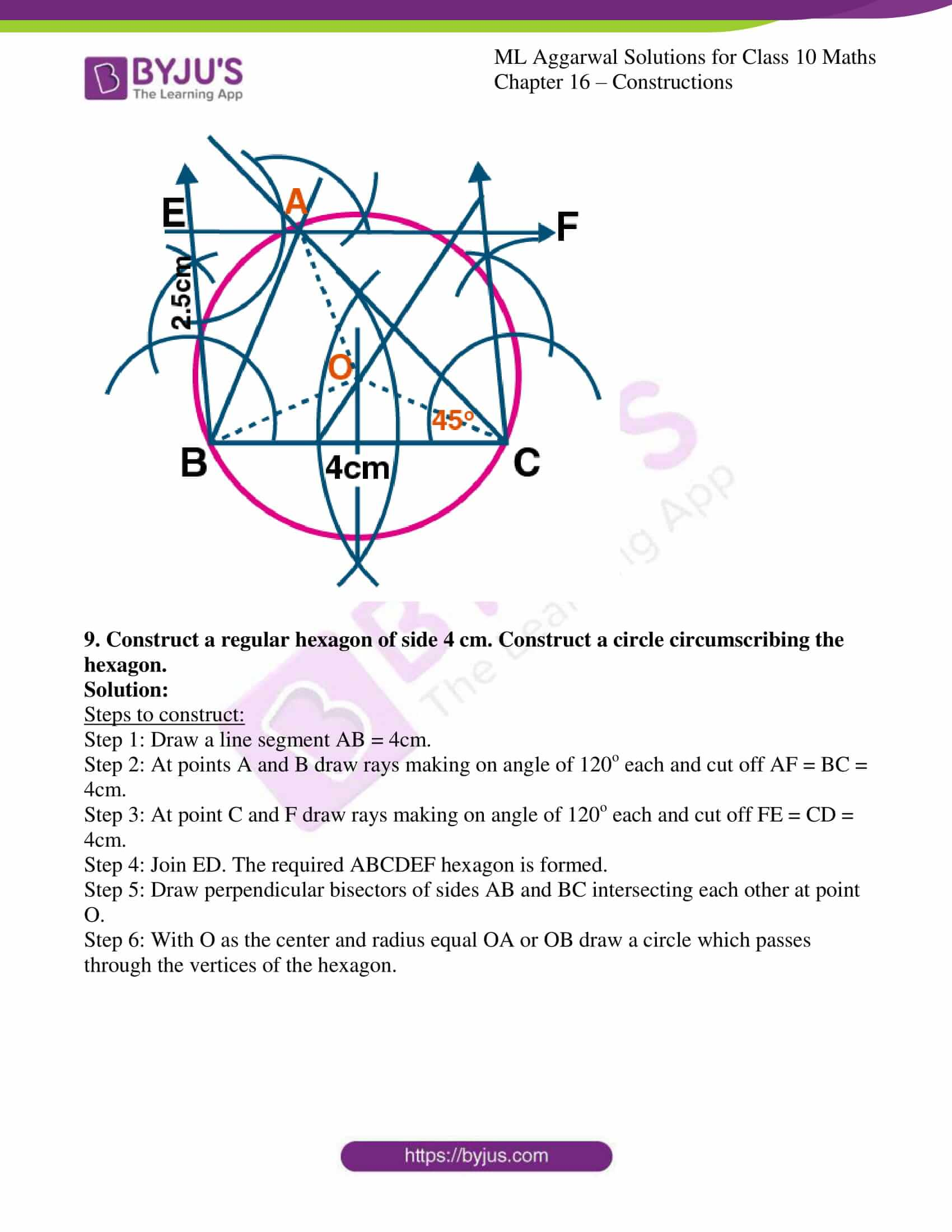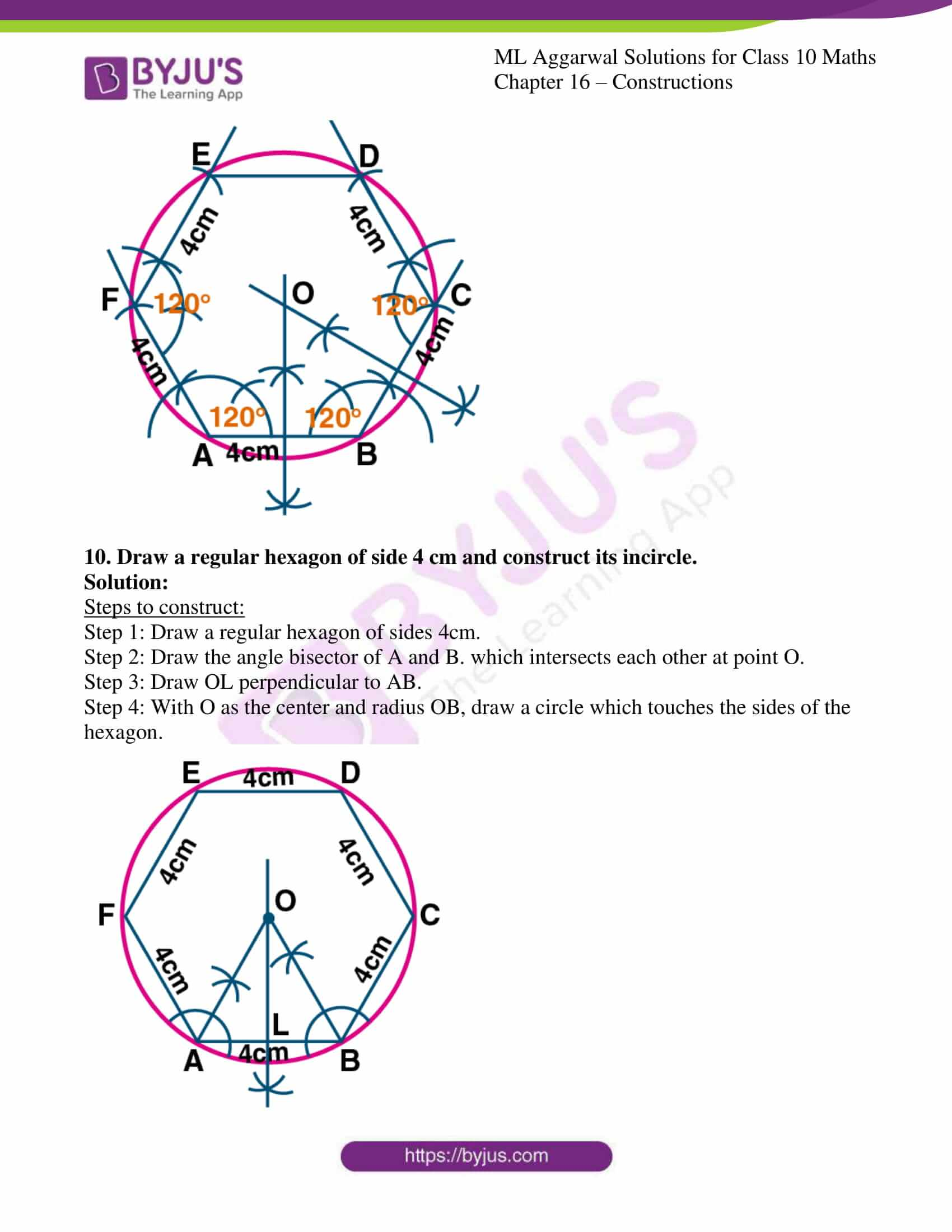### Access answers to ML Aggarwal Solutions for Class 10 Maths Chapter 16 – Constructions

EXERCISE 16.1

1. Use a ruler and compass only in this question.
(i) Draw a circle, centre O and radius 4 cm.
(ii) Mark a point P such that OP = 7 cm.
Construct the two tangents to the circle from P. Measure and record the length of one of the tangents.
Solution:

Steps to construct:

Step 1: Draw a circle with center O and radius 4cm and mark that point as A.

Step 2: Take a point P such that OP = 7cm.

Step 3: Bisect OB at M.

Step 4: With center M and diameter OP, draw another circle intersecting the given circle at A and B.

Step 5: Join PA and PB. Hence PA and PB are pair of tangents to the circle.

Step 6: On measuring PA, it is equal to 5.5cm.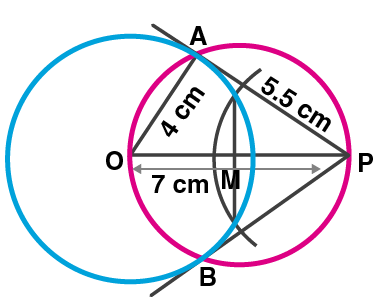2. Draw a line AB = 6 cm. Construct a circle with AB as diameter. Mark a point P at a distance of 5 cm from the mid-point of AB. Construct two tangents from P to the circle with AB as diameter. Measure the length of each tangent
Solution:

Steps to construct:

Step 1: Draw a line segment AB = 6cm.

Step 2: Draw its perpendicular bisector bisecting it at point O.

Step 3: With center O and radius OB, draw a circle.

Step 4: Extend AB to point P such that OP = 5cm.

Step 5: Draw its perpendicular bisector intersecting it at point M.

Step 6: With center M and radius OM, draw a circle which intersects the given circle at T and S.

Step 7: Join OT, OS, PT and PS. Hence, PT and PS are the required tangents to the given circle.

Step 8: On measuring each tangent is 4cm long. PT = PS = 4cm.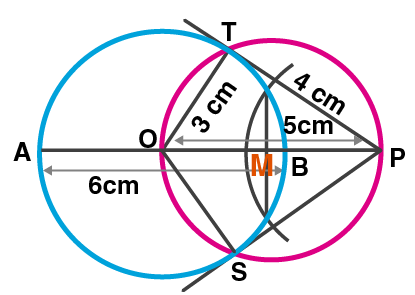3. Construct a tangent to a circle of radius 4cm from a point on the concentric circle of radius 6 cm and measure its length. Also, verify the measurement by actual calculation.
Solution:

Steps to construct:

Step 1: Mark a point O.

Step 2: With center O and radius 4cm and 6cm, draw two concentric circles.

Step 3: Join OA and mark its mid-point as M.

Step 4: With center M and radius MA, draw another circle which intersects the first circle at P and Q.

Step 5: Join AP and AQ. Hence, AP and AQ are the required tangents to the first circle from point A.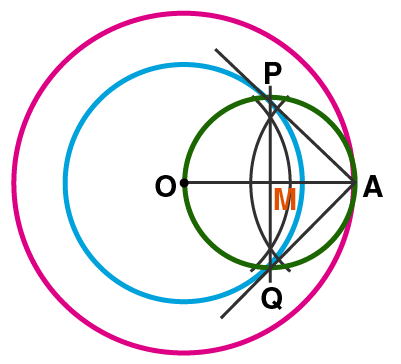4. Draw a circle of radius 3 cm. Take two points P and Q on one of its extended diameter each at a distance of 7 cm from its centre. Draw tangents to the circle from these two points P and Q.
Solution:

Steps to construct:

Step 1: Consider a point O on a line, with center O, and radius 3cm, draw a circle.

Step 2: Extend its diameters on both sides and cut off OP = OQ = 7cm.

Step 3: Mark the mid-points of OP and OQ as M and N respectively.

Step 4: With Centers M and N and OP and OQ as diameters, draw circles which intersect the given circle at A, B and C, D respectively.

Step 5: Join PA, PB, QC, QD. Hence, PA, PB and QC, QD are the required tangents.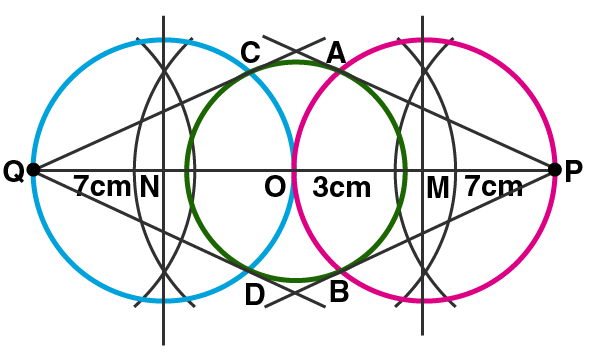5. Draw a line segment AB of length 8 cm. Taking A as centre, draw a circle of radius 4 cm and taking B as centre, draw another circle of radius 3 cm. Construct tangents to each circle from the centre of the other circle.
Solution:

Steps to construct:

Step 1: Draw a line segment AB = 8cm.

Step 2: With center as A and radius 4cm, with center as B and radius 3cm, draw circles.

Step 3: Draw the third circle AB as diameter which intersects the given two circles at C and D, P and Q respectively.

Step 4: Join AC, AD, BP, BQ. Hence, AC and AD, BP and BQ are the required tangents.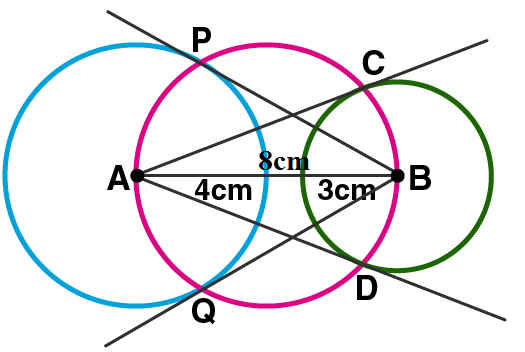EXERCISE 16.2

1. Draw an equilateral triangle of side 4 cm. Draw its circumcircle.
Solution:

Steps to construct:

Step 1: Draw a line segment BC = 4cm.

Step 2: With centers B and C, draw two arcs of radius 4cm which intersects each other at point A.

Step 3: Join AB and AC.

Step 4: Draw the right bisector of BC and AC intersecting each other at point O.

Step 5: Join OA, OB and OC.

Step 6: With center as O, and radius equal to OB or OC or OA, draw a circle which passes through points A, B and C.

Hence the required circumcircle of triangle ABC is given below.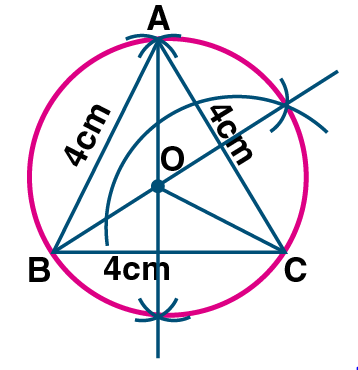2. Using a ruler and a pair of compasses only, construct:
(i) A triangle ABC given AB = 4 cm, BC = 6 cm and ∠ABC = 90°.
(ii) A circle which passes through the points A, B and C and mark its centre as O. (2008)
Solution:

Steps to construct:

Step 1: Draw a line segment AB = 4cm.

Step 2: At point B, draw a ray BX making an angle of 90o and cut off BC = 6cm.

Step 3: Join AC.

Step 4: Draw the perpendicular bisectors of sides AB and AC intersecting each other at point O.

Step 5: With center as O, and radius equal to OB or OA or OC, draw a circle which passes through points A, B, C.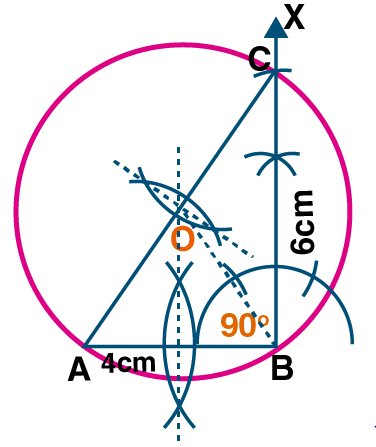3. Construct a triangle with sides 3 cm, 4 cm and 5 cm. Draw its circumcircle and measure its radius.
Solution:

Steps to construct:

Step 1: Draw a line segment BC = 4cm.

Step 2: With Center as B and radius 3cm, with center as C and radius 5cm draw two arcs which intersect each other at point A.

Step 3: Join AB and AC.

Step 4: Draw the perpendicular bisector of sides BC and AC which intersects each other at point O.

Step 5: Join OB.

Step 6: With center as O and radius OB, draw a circle which pass through A, B, C.

Step 7: On measuring the radius OB = 2.5cm.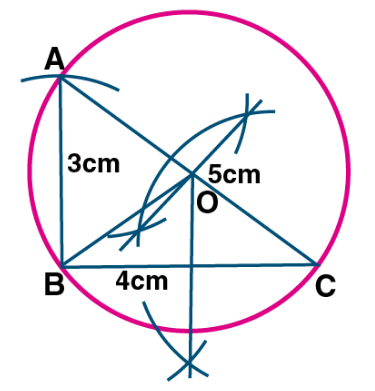4. Using a ruler and compasses only:
(i) Construe a triangle ABC with the following data:
Base AB = 6 cm, AC = 5.2 cm and ∠CAB = 60°.
(ii) In the same diagram, draw a circle which passes through the points A, B and C. and mark its centre O.
Solution:

Steps to construct:

Step 1: Draw a line segment AB = 6cm.

Step 2: At point A, draw a ray making an angle of 60o.

Step 3: With B as the center and radius 5.2cm, draw an arc which intersects the ray at C.

Step 4: Join BC.

Step 5: Draw the perpendicular bisector of sides AB and BC which intersects each other at point O.

Step 6: With center as O and radius OA, draw a circle which touches through the points A, B, C.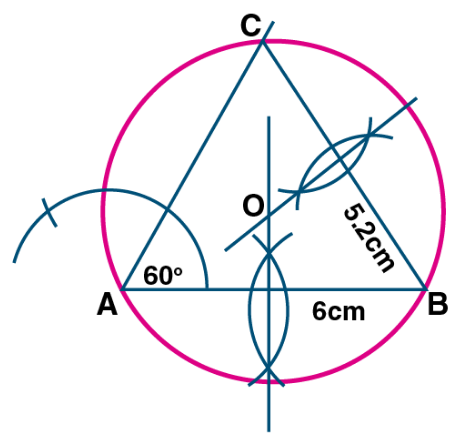5. Using ruler and compasses only, draw an equilateral triangle of side 5 cm and draw its inscribed circle. Measure the radius of the circle.
Solution:

Steps to construct:

Step 1: Draw a line segment BC = 5cm.

Step 2: With Center as B and radius 5cm, with center as C and radius 5cm draw two arcs which intersect each other at point A.

Step 3: Join AB and AC.

Step 4: Draw the angle bisector of angles B and C which intersects each other at point I.

Step 5: From I, draw a perpendicular ID on BC.

Step 6: With center as I and radius ID, draw a circle which touches the sides of the triangle internally.

Step 7: On measuring the radius ID = 1.5cm (approx).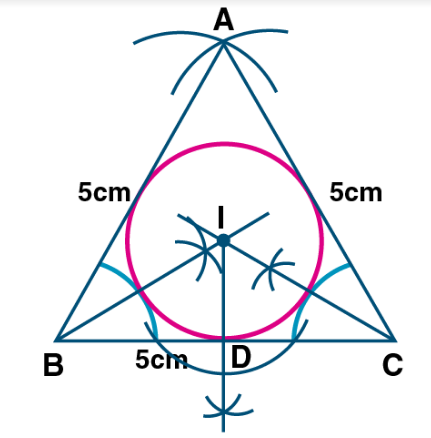6. (i) Conduct a triangle ABC with BC = 6.4 cm, CA = 5.8 cm and ∠ ABC = 60°. Draw its incircle. Measure and record the radius of the incircle.
(ii) Construct a ∆ABC with BC = 6.5 cm, AB = 5.5 cm, AC = 5 cm. Construct the incircle of the triangle. Measure and record the radius of the incircle. (2014)
Solution:

Steps to construct:

Step 1: Draw a line segment BC = 6.4cm.

Step 2: Construct an angle of 60o at point B.

Step 3: With C as center and radius CA = 5.8cm, draw an arc cutting BD at A.

Step 4: Join AC.

Step 5: Draw the angle bisector of angle B and angle C which intersect each other at point O.

Step 6: Draw OE perpendicular to BC, intersecting BC at point E.

Step 7: With O as the center and OE as the radius draw the required incircle.

Step 8: On measuring the radius OE = 1.5cm.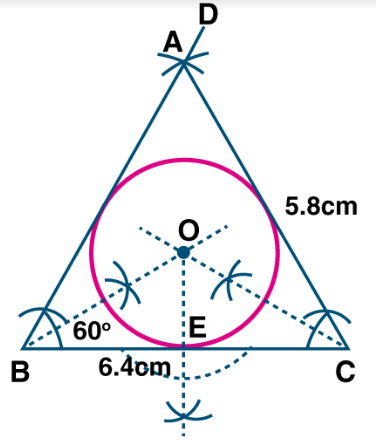Steps to construct:

Step 1: Draw a line segment BC = 6.5cm.

Step 2: With B as center and C as center draw arcs AB = 5.5cm and AC = 5cm.

Step 3: Join AB and AC.

Step 4: Draw the angle bisectors of B and C. The bisectors meet at point O.

Step 5: With O as the center. Draw an incircle which touches all the sides of the triangle ABC.

Step 6: From point O draw a perpendicular to side BC which cuts at point N.

Step 7: On measuring the radius ON = 1.5cm.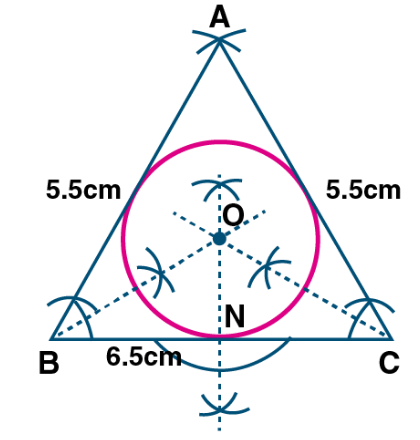7. The bisectors of angles A and B of a scalene triangle ABC meet at O.
(i) What is the point O called?
(ii) OR and OQ is drawn a perpendicular to AB and CA respectively. What is the relation between OR and OQ?
(iii) What is the relation between ∠ACO and ∠BCO?
Solution:

(i) The point O where the angle bisectors meet is called the incenter of the triangle.

(ii) The perpendicular drawn from point O to AB and CA are equal. i.e., OR and OQ.

(iii) ∠ACO = ∠BCO. OC will bisect the ∠C.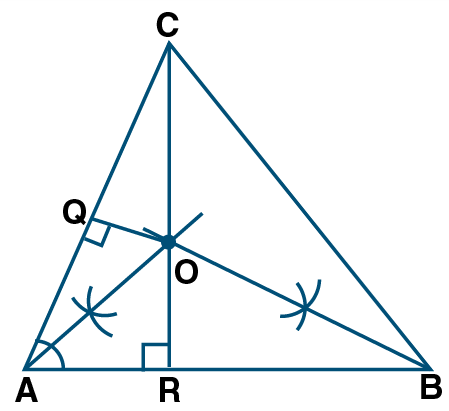8. Using ruler and compasses only, construct a triangle ABC in which BC = 4 cm, ∠ACB = 45° and the perpendicular from A on BC is 2.5 cm. Draw the circumcircle of triangle ABC and measure its radius.
Solution:

Steps to construct:

Step 1: Draw a line segment BC = 4cm.

Step 2: At point B, draw a perpendicular and cut off BE = 2.5cm.

Step 3: From, E, draw a line EF parallel to BC.

Step 4: From point C, draw a ray making an angle 45o which intersects EF at point A.

Step 5: Join AB.

Step 6: Draw the perpendicular bisectors of sides BC and AC intersecting each other at point O.

Step 7: Join OB, OC and OA.

Step 8: With O as the center and radius OB or OC or OA draw a circle which passes through points A, B, C.

Step 9: On measuring the radius OB = 2cm.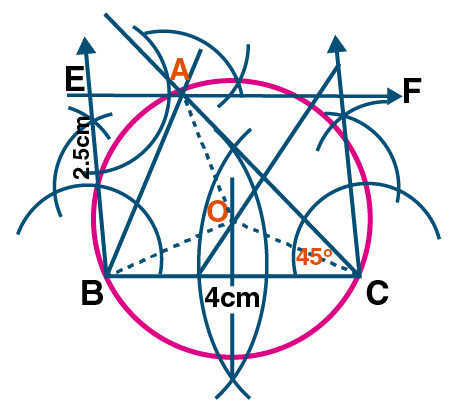9. Construct a regular hexagon of side 4 cm. Construct a circle circumscribing the hexagon.
Solution:

Steps to construct:

Step 1: Draw a line segment AB = 4cm.

Step 2: At points A and B draw rays making on angle of 120o each and cut off AF = BC = 4cm.

Step 3: At point C and F draw rays making on angle of 120o each and cut off FE = CD = 4cm.

Step 4: Join ED. The required ABCDEF hexagon is formed.

Step 5: Draw perpendicular bisectors of sides AB and BC intersecting each other at point O.

Step 6: With O as the center and radius equal OA or OB draw a circle which passes through the vertices of the hexagon.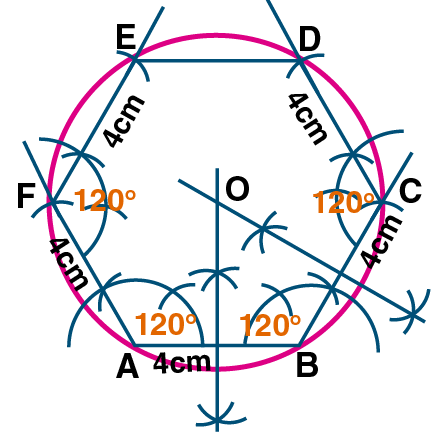10. Draw a regular hexagon of side 4 cm and construct its incircle.
Solution:

Steps to construct:

Step 1: Draw a regular hexagon of sides 4cm.

Step 2: Draw the angle bisector of A and B. which intersects each other at point O.

Step 3: Draw OL perpendicular to AB.

Step 4: With O as the center and radius OB, draw a circle which touches the sides of the hexagon.